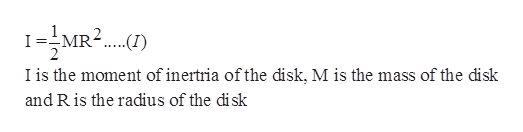# Torque and Force How much torque do you need to apply to a 1kg disk of radius 10cm to get an angular acceleration of 3 rads/s2. How much force do you need to exert on the edge of the disk to achieve this torque? Assume the disk is rotating about an axis through its center perpendicular to its face, and you are applying the force perpendicular to the radius of the desk, as you were spinning thre disk with your hand.

Question
41 views

Torque and Force

How much torque do you need to apply to a 1kg disk of radius 10cm to get an angular acceleration of 3 rads/s2. How much force do you need to exert on the edge of the disk to achieve this torque? Assume the disk is rotating about an axis through its center perpendicular to its face, and you are applying the force perpendicular to the radius of the desk, as you were spinning thre disk with your hand.

check_circle

Step 1

The disk is rotating about an axis through its center perpendicular to the face of the disk.

Step 2

The equation for the moment of inertia of a disk rotating about an axis perpendicular to the face of the disk is:help_outlineImage TranscriptioncloseIMR2.) I is the moment of inertria of the disk, M is the mass of the disk and R is the radius of the di sk fullscreen
Step 3

Substitute 1kg for M and 10cm ...

### Want to see the full answer?

See Solution

#### Want to see this answer and more?

Solutions are written by subject experts who are available 24/7. Questions are typically answered within 1 hour.*

See Solution
*Response times may vary by subject and question.
Tagged in

### Physics